# Hypothesis Testing

using computer simulation. Based on examples from the infer package. Code for Quiz 13.

Load the R packages we will use.

``````library(tidyverse)
library(infer)
library(skimr)
``````
• Replace all the instances of ???. These are answers on your moodle quiz.

• Run all the individual code chunks to make sure the answers in this file correspond with your quiz answers

• After you check all your code chunks run then you can knit it. It won’t knit until the ??? are replaced

• Save a plot to be your preview plot

## Question: t-test

• The data this quiz uses is a subset of `HR`

• Look at the variable definitions

• Note that the variables evaluation and salary have been recoded to be represented as words instead of numbers

• Set random seed generator to 123

``````set.seed(123)
``````

hr_2_tidy.csv is the name of your data subset

• Read it into and assign to `hr`

• Note: col_types = “fddfff” defines the column types factor-double-double-factor-factor-factor
``````hr  <- read_csv("https://estanny.com/static/week13/data/hr_2_tidy.csv",
col_types = "fddfff")
``````

use `skim` to summarize the data in `hr`

``````skim(hr)
``````
 Name hr Number of rows 500 Number of columns 6 _______________________ Column type frequency: factor 4 numeric 2 ________________________ Group variables None

Variable type: factor

skim_variable n_missing complete_rate ordered n_unique top_counts
gender 0 1 FALSE 2 mal: 256, fem: 244
evaluation 0 1 FALSE 4 bad: 154, fai: 142, goo: 108, ver: 96
salary 0 1 FALSE 6 lev: 95, lev: 94, lev: 87, lev: 85
status 0 1 FALSE 3 fir: 194, pro: 179, ok: 127

Variable type: numeric

skim_variable n_missing complete_rate mean sd p0 p25 p50 p75 p100 hist
age 0 1 39.86 11.55 20.3 29.60 40.2 50.1 59.9 ▇▇▇▇▇
hours 0 1 49.39 13.15 35.0 37.48 45.6 58.9 79.9 ▇▃▂▂▂

The mean hours worked per week is: 49.4

## Q: Is the mean number of hours worked per week 48?

`specify` that `hours` is the variable of interest

``````hr  %>%
specify(response = hours)
``````
``````Response: hours (numeric)
# A tibble: 500 × 1
hours
<dbl>
1  78.1
2  35.1
3  36.9
4  38.5
5  36.1
6  78.1
7  76
8  35.6
9  35.6
10  56.8
# … with 490 more rows``````

`hypothesize` that the average hours worked is 48

``````hr  %>%
specify(response = hours)  %>%
hypothesize(null = "point", mu = 48)
``````
``````Response: hours (numeric)
Null Hypothesis: point
# A tibble: 500 × 1
hours
<dbl>
1  78.1
2  35.1
3  36.9
4  38.5
5  36.1
6  78.1
7  76
8  35.6
9  35.6
10  56.8
# … with 490 more rows``````

`generate` 1000 replicates representing the null hypothesis

``````hr %>%
specify(response = hours)  %>%
hypothesize(null = "point", mu = 48)  %>%
generate(reps = 1000, type = "bootstrap")
``````
``````Response: hours (numeric)
Null Hypothesis: point
# A tibble: 500,000 × 2
# Groups:   replicate [1,000]
replicate hours
<int> <dbl>
1         1  39.7
2         1  44.3
3         1  46.8
4         1  33.7
5         1  39.6
6         1  39.5
7         1  40.5
8         1  55.8
9         1  72.6
10         1  35.7
# … with 499,990 more rows``````

The output has 500,000 rows

`calculate` the distribution of statistics from the generated data

• Assign the output null_t_distribution

• Display null_t_distribution

``````null_t_distribution  <- hr  %>%
specify(response = age)  %>%
hypothesize(null = "point", mu = 48)  %>%
generate(reps = 1000, type = "bootstrap")  %>%
calculate(stat = "t")

null_t_distribution
``````
``````Response: age (numeric)
Null Hypothesis: point
# A tibble: 1,000 × 2
replicate     stat
<int>    <dbl>
1         1  0.144
2         2 -1.72
3         3  0.404
4         4 -1.11
5         5  0.00894
6         6  1.46
7         7 -0.905
8         8 -0.663
9         9  0.291
10        10  3.09
# … with 990 more rows``````

null_t_distribution has 1000 t-stats

visualize the simulated null distribution

``````visualize(null_t_distribution)
``````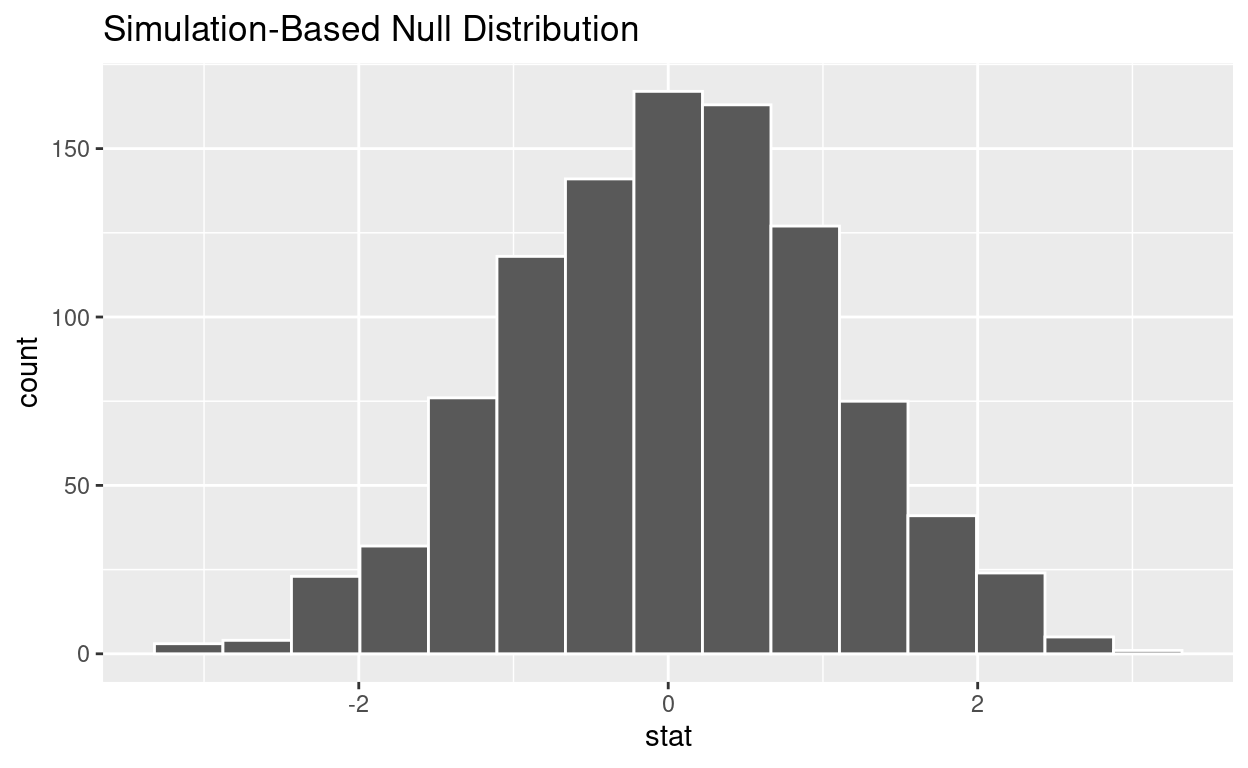calculate the statistic from your observed data

• Assign the output observed_t_statistic

• Display observed_t_statistic

``````observed_t_statistic  <- hr  %>%
specify(response = hours)  %>%
hypothesize(null = "point", mu = 48)  %>%
calculate(stat = "t")

observed_t_statistic
``````
``````Response: hours (numeric)
Null Hypothesis: point
# A tibble: 1 × 1
stat
<dbl>
1  2.37``````

get_p_value from the simulated null distribution and the observed statistic

``````null_t_distribution  %>%
get_p_value(obs_stat = observed_t_statistic, direction = "two-sided")
``````
``````# A tibble: 1 × 1
p_value
<dbl>
1   0.014``````

shade_p_value on the simulated null distribution

``````null_t_distribution  %>%
visualize() +
shade_p_value(obs_stat = observed_t_statistic, direction = "two-sided")
``````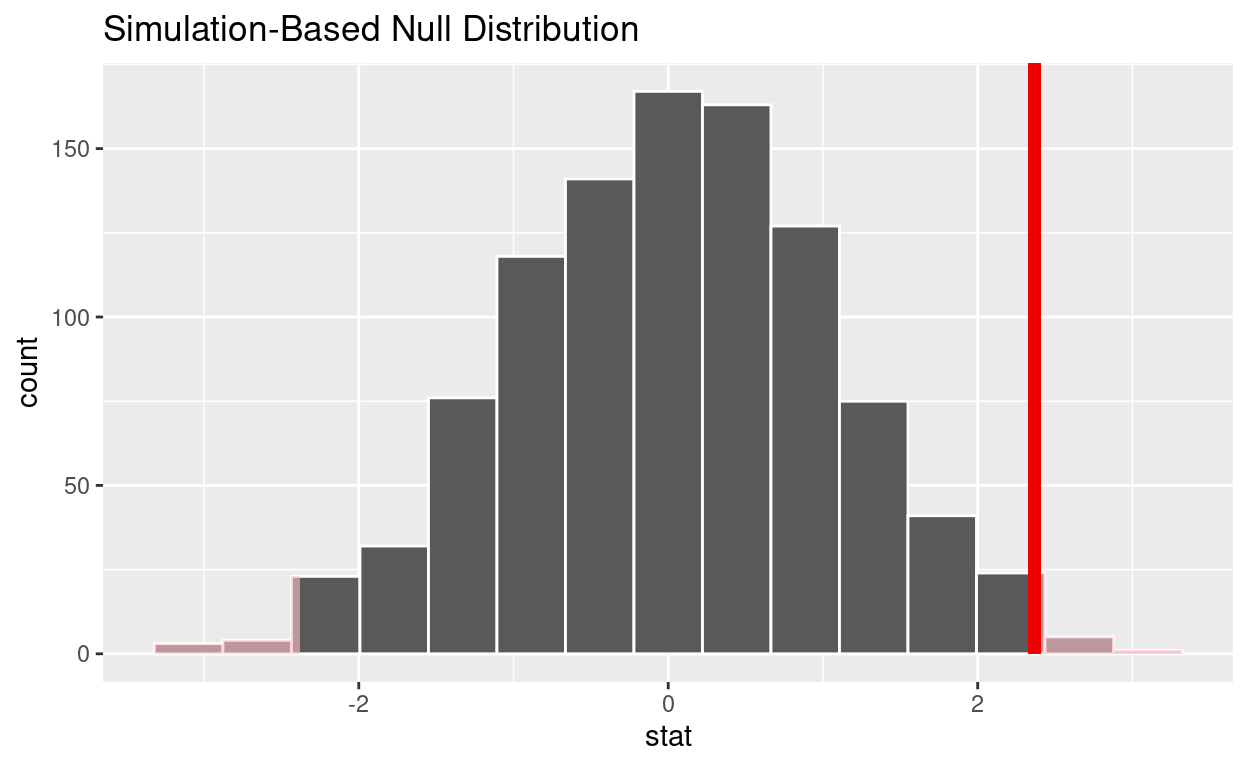Is the p-value < 0.05? yes

Does your analysis support the null hypothesis that the true mean number of hours worked was 48? no

## Question: 2 sample t-test

hr_3_tidy.csv is the name of your data subset

• Read it into and assign to hr_2

• Note: col_types = “fddfff” defines the column types factor-double-double-factor-factor-factor
``````hr_2 <- read_csv("https://estanny.com/static/week13/data/hr_3_tidy.csv",
col_types = "fddfff")
``````

## Q: Is the average number of hours worked the same for both genders?

use skim to summarize the data in hr_2 by gender

``````hr_2 %>%
group_by(gender)  %>%
skim()
``````
 Name Piped data Number of rows 500 Number of columns 6 _______________________ Column type frequency: factor 3 numeric 2 ________________________ Group variables gender

Variable type: factor

skim_variable gender n_missing complete_rate ordered n_unique top_counts
evaluation male 0 1 FALSE 4 bad: 72, fai: 67, goo: 61, ver: 47
evaluation female 0 1 FALSE 4 bad: 76, fai: 71, goo: 61, ver: 45
salary male 0 1 FALSE 6 lev: 47, lev: 43, lev: 43, lev: 42
salary female 0 1 FALSE 6 lev: 51, lev: 46, lev: 45, lev: 43
status male 0 1 FALSE 3 fir: 98, pro: 81, ok: 68
status female 0 1 FALSE 3 fir: 98, pro: 91, ok: 64

Variable type: numeric

skim_variable gender n_missing complete_rate mean sd p0 p25 p50 p75 p100 hist
age male 0 1 38.23 10.86 20 28.9 37.9 47.05 59.9 ▇▇▇▇▅
age female 0 1 40.56 11.67 20 31.0 40.3 50.50 59.8 ▆▆▇▆▇
hours male 0 1 49.55 13.11 35 38.4 45.4 57.65 79.9 ▇▃▂▂▂
hours female 0 1 49.80 13.38 35 38.2 45.6 59.40 79.8 ▇▂▃▂▂
• Females worked an average of 49.8 hours per week

• Males worked an average of 49.6 hours per week

Use geom_boxplot to plot distributions of hours worked by gender

``````hr_2 %>%
ggplot(aes(x = gender, y = hours)) +
geom_boxplot()
``````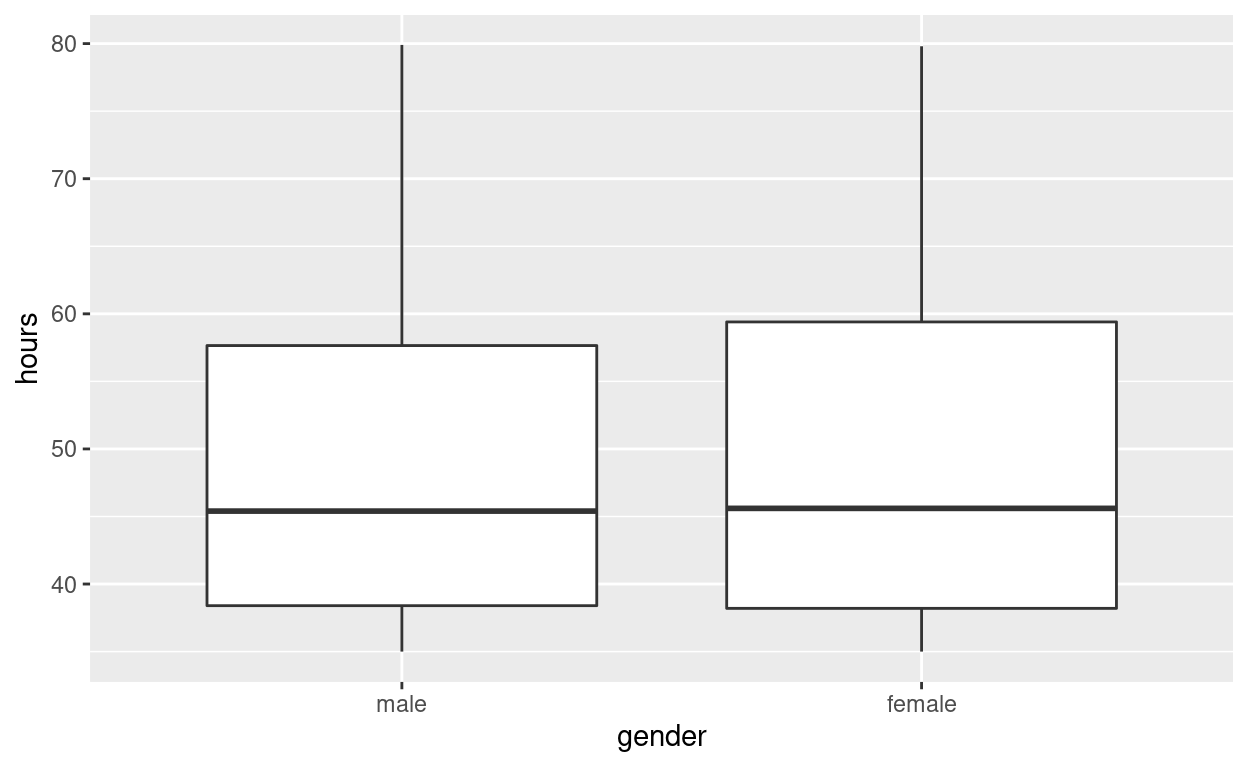specify the variables of interest are hours and gender

``````hr_2 %>%
specify(response = hours, explanatory = gender)
``````
``````Response: hours (numeric)
Explanatory: gender (factor)
# A tibble: 500 × 2
hours gender
<dbl> <fct>
1  49.6 male
2  39.2 female
3  63.2 female
4  42.2 male
5  54.7 male
6  54.3 female
7  37.3 female
8  45.6 female
9  35.1 female
10  53   male
# … with 490 more rows``````

hypothesize that the number of hours worked and gender are independent

``````hr_2  %>%
specify(response = hours, explanatory = gender)  %>%
hypothesize(null = "independence")
``````
``````Response: hours (numeric)
Explanatory: gender (factor)
Null Hypothesis: independence
# A tibble: 500 × 2
hours gender
<dbl> <fct>
1  49.6 male
2  39.2 female
3  63.2 female
4  42.2 male
5  54.7 male
6  54.3 female
7  37.3 female
8  45.6 female
9  35.1 female
10  53   male
# … with 490 more rows``````

generate 1000 replicates representing the null hypothesis

``````hr_2 %>%
specify(response = hours, explanatory = gender)  %>%
hypothesize(null = "independence")  %>%
generate(reps = 1000, type = "permute")
``````
``````Response: hours (numeric)
Explanatory: gender (factor)
Null Hypothesis: independence
# A tibble: 500,000 × 3
# Groups:   replicate [1,000]
hours gender replicate
<dbl> <fct>      <int>
1  55.7 male           1
2  35.5 female         1
3  35.1 female         1
4  44.2 male           1
5  52.8 male           1
6  46   female         1
7  41.2 female         1
8  52.9 female         1
9  35.6 female         1
10  35   male           1
# … with 499,990 more rows``````

The output has *500,000** rows

calculate the distribution of statistics from the generated data

• Assign the output `null_distribution_2_sample_permute`

• Display `null_distribution_2_sample_permute`

``````null_distribution_2_sample_permute  <- hr_2 %>%
specify(response = hours, explanatory = gender)  %>%
hypothesize(null = "independence")  %>%
generate(reps = 1000, type = "permute")  %>%
calculate(stat = "t", order = c("female", "male"))

null_distribution_2_sample_permute
``````
``````Response: hours (numeric)
Explanatory: gender (factor)
Null Hypothesis: independence
# A tibble: 1,000 × 2
replicate    stat
<int>   <dbl>
1         1 -1.81
2         2 -1.29
3         3  0.0525
4         4 -0.793
5         5  0.826
6         6  0.429
7         7  0.0843
8         8 -0.264
9         9  2.42
10        10  0.603
# … with 990 more rows``````
• null_t_distribution has 1000 t-stats

visualize the simulated null distribution

``````visualize(null_distribution_2_sample_permute)
``````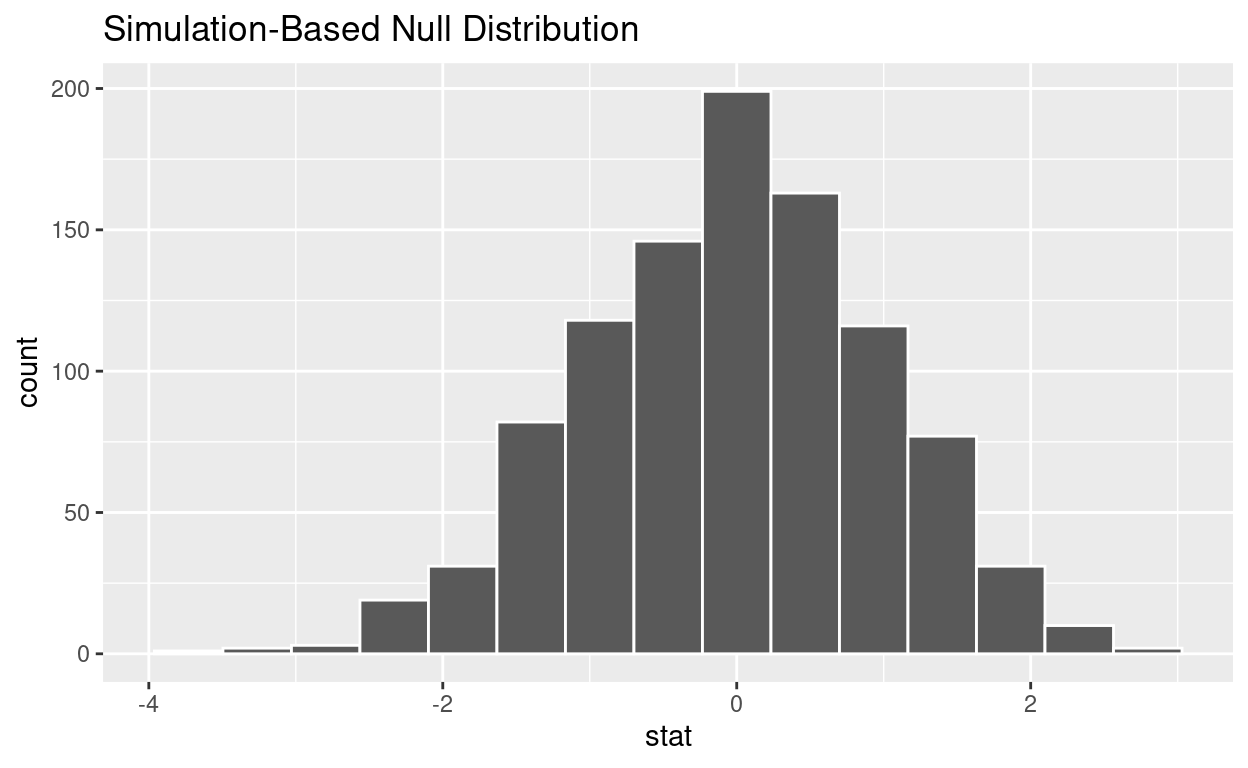calculate the statistic from your observed data

• Assign the output observed_t_2_sample_stat

• Display observed_t_2_sample_stat

``````observed_t_2_sample_stat  <- hr_2 %>%
specify(response = hours, explanatory = gender)  %>%
calculate(stat = "t", order = c("female", "male"))
observed_t_2_sample_stat
``````
``````Response: hours (numeric)
Explanatory: gender (factor)
# A tibble: 1 × 1
stat
<dbl>
1 0.208``````

get_p_value from the simulated null distribution and the observed statistic

``````null_t_distribution  %>%
get_p_value(obs_stat = observed_t_2_sample_stat, direction = "two-sided")
``````
``````# A tibble: 1 × 1
p_value
<dbl>
1   0.878``````

shade_p_value on the simulated null distribution

``````null_t_distribution  %>%
visualize() +
shade_p_value(obs_stat = observed_t_2_sample_stat, direction = "two-sided")
``````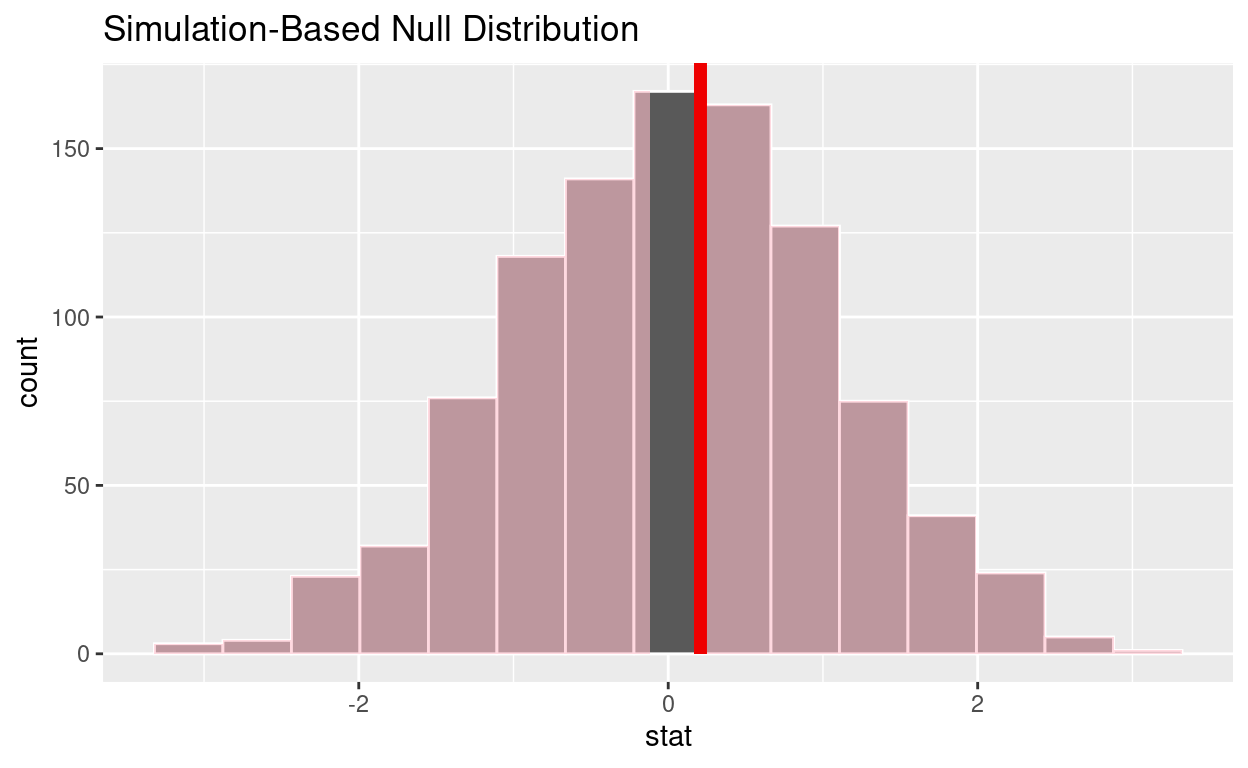Is the p-value < 0.05? no

Does your analysis support the null hypothesis that the true mean number of hours worked by female and male employees was the same? yes

## Question: ANOVA

hr_2_tidy.csv is the name of your data subset

• Read it into and assign to hr_anova

• Note: col_types = “fddfff” defines the column types factor-double-double-factor-factor-factor

``````hr_anova <- read_csv("https://estanny.com/static/week13/data/hr_2_tidy.csv",
col_types = "fddfff")
``````

## Q: Is the average number of hours worked the same for all three status (fired, ok and promoted) ?

use skim to summarize the data in hr_anova by status

``````hr_anova %>%
group_by(status)  %>%
skim()
``````
 Name Piped data Number of rows 500 Number of columns 6 _______________________ Column type frequency: factor 3 numeric 2 ________________________ Group variables status

Variable type: factor

skim_variable status n_missing complete_rate ordered n_unique top_counts
gender promoted 0 1 FALSE 2 mal: 90, fem: 89
gender fired 0 1 FALSE 2 fem: 101, mal: 93
gender ok 0 1 FALSE 2 mal: 73, fem: 54
evaluation promoted 0 1 FALSE 4 goo: 70, ver: 62, fai: 24, bad: 23
evaluation fired 0 1 FALSE 4 bad: 78, fai: 72, goo: 25, ver: 19
evaluation ok 0 1 FALSE 4 bad: 53, fai: 46, ver: 15, goo: 13
salary promoted 0 1 FALSE 6 lev: 42, lev: 42, lev: 39, lev: 34
salary fired 0 1 FALSE 6 lev: 54, lev: 44, lev: 34, lev: 24
salary ok 0 1 FALSE 6 lev: 32, lev: 31, lev: 26, lev: 19

Variable type: numeric

skim_variable status n_missing complete_rate mean sd p0 p25 p50 p75 p100 hist
age promoted 0 1 40.63 11.25 20.4 30.75 41.10 50.25 59.9 ▆▇▇▇▇
age fired 0 1 40.03 11.53 20.3 29.45 40.40 50.08 59.9 ▇▅▇▆▆
age ok 0 1 38.50 11.98 20.3 28.15 38.70 49.45 59.9 ▇▆▅▅▆
hours promoted 0 1 59.21 12.66 35.0 49.75 58.90 70.65 79.9 ▅▆▇▇▇
hours fired 0 1 41.67 8.37 35.0 36.10 38.45 43.40 77.7 ▇▂▁▁▁
hours ok 0 1 47.35 10.86 35.0 37.10 45.70 54.50 78.9 ▇▅▃▂▁
• Employees that were fired worked an average of 40.0 hours per week

• Employees that were ok worked an average of 38.5 hours per week

• Employees that were promoted worked an average of 59.2 hours per week

Use geom_boxplot to plot distributions of hours worked by status

``````hr_anova %>%
ggplot(aes(x = status, y = hours)) +
geom_boxplot()
``````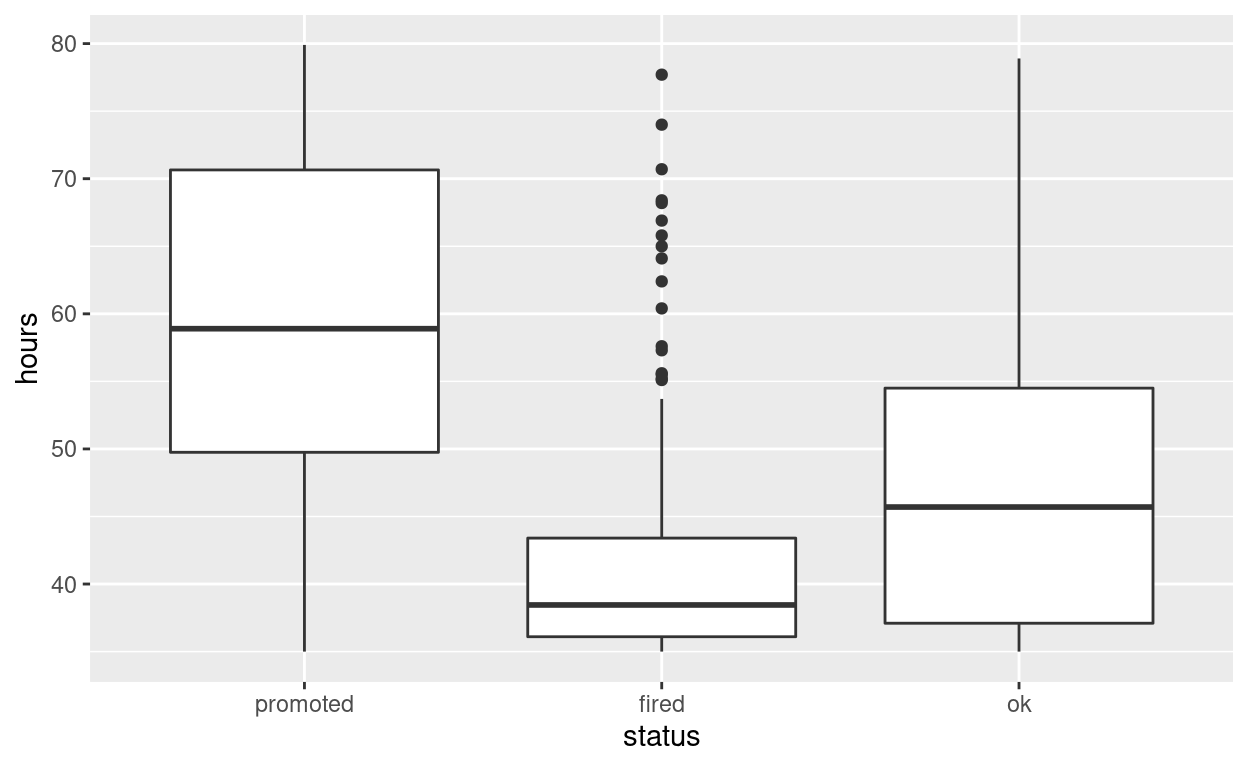specify the variables of interest are hours and status

``````hr_anova %>%
specify(response = hours, explanatory = status)
``````
``````Response: hours (numeric)
Explanatory: status (factor)
# A tibble: 500 × 2
hours status
<dbl> <fct>
1  78.1 promoted
2  35.1 fired
3  36.9 fired
4  38.5 fired
5  36.1 fired
6  78.1 promoted
7  76   promoted
8  35.6 fired
9  35.6 ok
10  56.8 promoted
# … with 490 more rows``````

hypothesize that the number of hours worked and status are independent

``````hr_anova  %>%
specify(response = hours, explanatory = status)  %>%
hypothesize(null = "independence")
``````
``````Response: hours (numeric)
Explanatory: status (factor)
Null Hypothesis: independence
# A tibble: 500 × 2
hours status
<dbl> <fct>
1  78.1 promoted
2  35.1 fired
3  36.9 fired
4  38.5 fired
5  36.1 fired
6  78.1 promoted
7  76   promoted
8  35.6 fired
9  35.6 ok
10  56.8 promoted
# … with 490 more rows``````

generate 1000 replicates representing the null hypothesis

``````hr_anova %>%
specify(response = hours, explanatory = status)  %>%
hypothesize(null = "independence")  %>%
generate(reps = 1000, type = "permute")
``````
``````Response: hours (numeric)
Explanatory: status (factor)
Null Hypothesis: independence
# A tibble: 500,000 × 3
# Groups:   replicate [1,000]
hours status   replicate
<dbl> <fct>        <int>
1  41.9 promoted         1
2  36.7 fired            1
3  35   fired            1
4  58.9 fired            1
5  36.1 fired            1
6  39.4 promoted         1
7  54.3 promoted         1
8  59.2 fired            1
9  40.2 ok               1
10  35.3 promoted         1
# … with 499,990 more rows``````

The output has 500,000 rows

calculate the distribution of statistics from the generated data

• Assign the output null_distribution_anova

• Display null_distribution_anova

``````null_distribution_anova  <- hr_anova %>%
specify(response = hours, explanatory = status)  %>%
hypothesize(null = "independence")  %>%
generate(reps = 1000, type = "permute")  %>%
calculate(stat = "F")

null_distribution_anova
``````
``````Response: hours (numeric)
Explanatory: status (factor)
Null Hypothesis: independence
# A tibble: 1,000 × 2
replicate  stat
<int> <dbl>
1         1 0.312
2         2 2.85
3         3 0.369
4         4 0.142
5         5 0.511
6         6 2.73
7         7 1.06
8         8 0.171
9         9 0.310
10        10 1.11
# … with 990 more rows``````

null_distribution_anova has 1000 F-stats

visualize the simulated null distribution

``````visualize(null_distribution_anova)
``````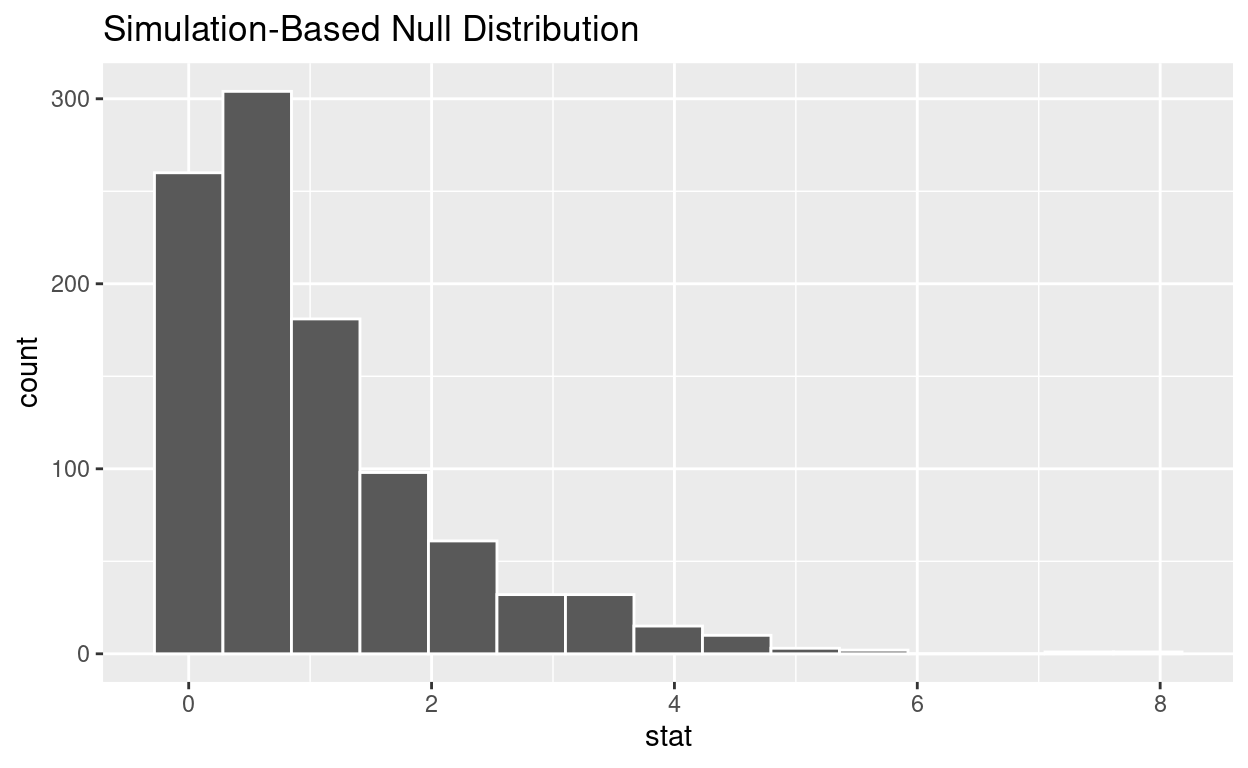calculate the statistic from your observed data

• Assign the output observed_f_sample_stat

• Display observed_f_sample_stat

``````observed_f_sample_stat  <- hr_anova %>%
specify(response = hours, explanatory = status)  %>%
calculate(stat = "F")

observed_f_sample_stat
``````
``````Response: hours (numeric)
Explanatory: status (factor)
# A tibble: 1 × 1
stat
<dbl>
1  128.``````

get_p_value from the simulated null distribution and the observed statistic

``````null_distribution_anova  %>%
get_p_value(obs_stat = observed_f_sample_stat, direction = "greater")
``````
``````# A tibble: 1 × 1
p_value
<dbl>
1       0``````

shade_p_value on the simulated null distribution

``````null_t_distribution  %>%
visualize() +
shade_p_value(obs_stat = observed_f_sample_stat, direction = "greater")
``````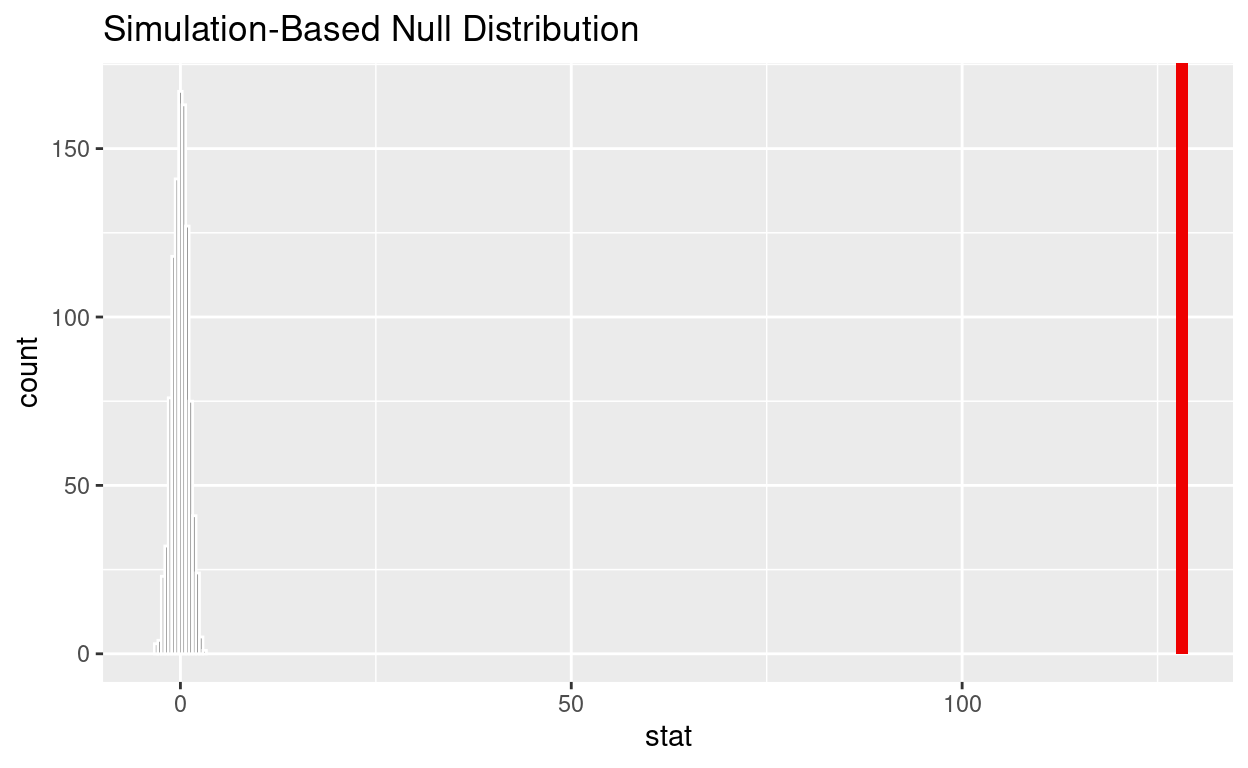Save the previous plot to preview.png and add to the yaml chunk at the top

``````ggsave(filename = "preview.png",
path = here::here("_posts", "2022-05-03-hypothesis-testing"))
``````

If the p-value < 0.05? yes

Does your analysis support the null hypothesis that the true means of the number of hours worked for those that were “fired”, “ok” and “promoted” were the same? no